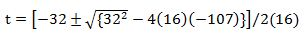# Respond to the Following Algebraic Expressions and Problems

User Generated

avan41

Mathematics

### Question Description

1.A ball is thrown downward from a window in building. Position at time t in seconds is s=16t^2+32t, where s is in feet. How long (to nearest tenth) take the ball to fall 107 feet?

2. Write in slope-intercept (-4,-5), m=2

3. Product (8p-1)(64p^2+8p+1)Student has agreed that all tutoring, explanations, and answers provided by the tutor will be used to help in the learning process and in accordance with Studypool's honor code & terms of service.1.A ball is thrown downward from a window in building. Position at time t in seconds is s=16t^2+32t, where s is in feet. How long (to nearest tenth) take the ball to fall 107 feet?

The equation is:

16t^2 + 32t = 107

16t^2 + 32t - 107 = 0

Find t using the quadratic formula: a = 16; b = 32; c = -107t = 1.385

2. Write in slope-intercept (-4,-5), m=2

Slope Intercept form:

y = mx+c

-5 = 2(-4) + c

-5 = -8 + c

c = 8-5

c = 3

Product (8p-1)(64p^2+8p+1)

= 8p(64p^2+8p+1) -1(64p^2+8p+1)

= 512 p^3 + 64p^2 +8p - 64p^2 - 8p + 1

= 512p^3 +1Npnqrzvpurycpragre (761)
Boston CollegeAnonymous
Just what I was looking for! Super helpful.Studypool4.7Trustpilot4.5Sitejabber4.4